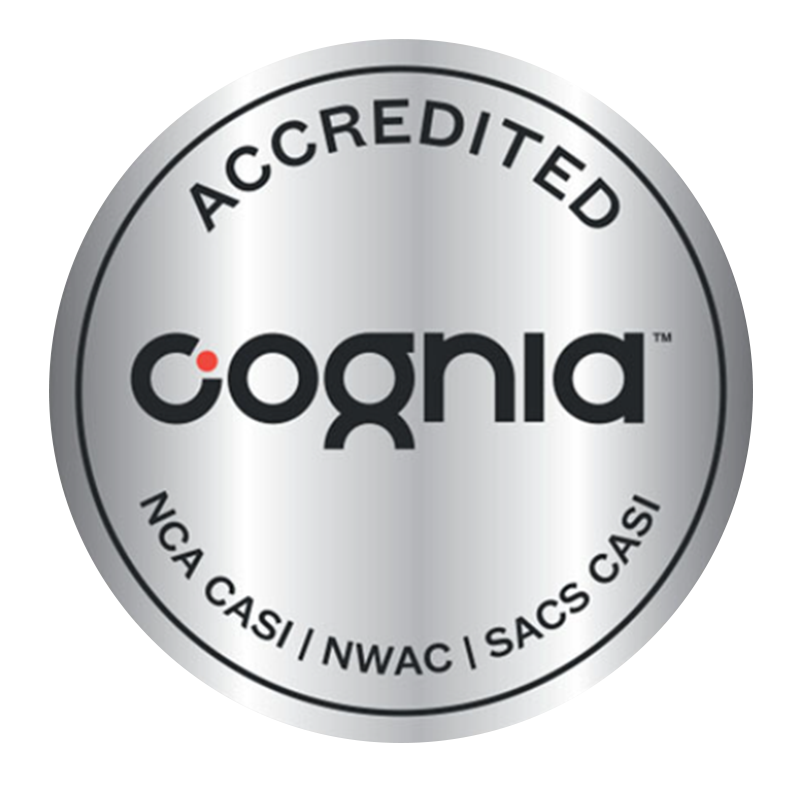Duration
34 Weeks
Requirements
Knowledge of the content of the Cambridge IGCSE is assumed.
Outcome
At the end of the first year of A Level, students have the option of writing AS Level exams
Suggested for
18-year-old students
Textbook List
View textbook list
Course Brochure

## Description

This course helps students develop a set of transferable skills. These include working with mathematical information, as well as the ability to think logically and independently, consider accuracy, model situations mathematically, analyse results and reflect on findings.

Students can apply these skills across a wide range of subjects and these skills equip them effectively for progression to higher education or directly into employment. At A Level, teachers can also choose from different routes depending on the choice of applied mathematics (Mechanics and/or Probability and Statistics).

### The A Level Mathematics Statistics course covers the following topics:

• Hypothesis Testing
• Critical Values
• Tails
• Errors
• Poisson Introduction
• Poisson Distribution
• Summing Poisson Distributions
• Poisson Approximation to the Binomial
• Normal Approximation to the Poisson
• Hypothesis Testing with the Poisson
• Probability Density Functions
• Features of PDFs
• Expectation and Variance of a Function
• Combining Independent Random Variables
• Working with More Than 2 Variables
• Linear Combinations of Variables
• Sampling
• Hypothesis Testing with the Normal
• Central Limit Theorem
• Confidence Intervals
• Central Limit Theorem and Confidence Intervals

## Fees

CambriLearn offers two packages, Premium and Standard. Our courses are priced in US dollars. You are welcome to pay in monthly instalments (10x instalments) or as a once-off annual fee.

If you need more advice, or would like to discuss your individual learning requirements, one of our education consultants can assist you with a formal quote once they have assessed your individual needs.

Save over 15%

Over 10 months

Save over 15%

Over 10 months